# A SEMI ANALYTICAL METHOD TO SOLVE J-INTEGRAL FOR MODE-I CRACK COMPONENTS

Applied Mechanics and Structure Safety Key Laboratory of Sichuan Province,School of Mechanics and Engineering,Southwest Jiaotong University,Chengdu 610031,China

Abstract

Keywords： J-integral ; plastic fracture ; semi-analytical formula ; energy equivalence hypothesis ; mode I crack

0

He Yi, Cai Lixun, Chen Hui, Peng Yunqiang. A SEMI ANALYTICAL METHOD TO SOLVE J-INTEGRAL FOR MODE-I CRACK COMPONENTS[J]. Chinese Journal of Theoretical and Applied Mechanics, 2018, 50(3): 579-588 https://doi.org/10.6052/0459-1879-18-026

## 引言

$J = J_{\rm e} + J_{\rm p}$(1)

$J_{\rm e} = f_1 (a_{\rm e} )\dfrac{P^2}{{E}'}$(2)

$J_{\rm p}= \alpha \sigma _0 \varepsilon _0 ch_1 \left( {\dfrac{P}{P_0 }}\right)^{n + 1}$(3)

$J_{\rm e} = \dfrac{K^2}{{E}'} (4)$

$J_{\rm p} = \eta \dfrac{U_{\rm p} }{B_{\rm N} b}$(5)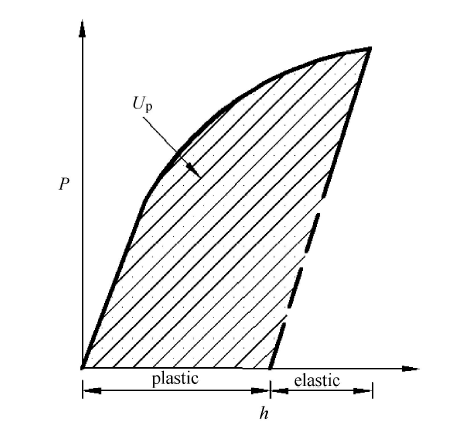Fig. 1   The schematic diagram of plastic work

$J = - \Big(\dfrac{\partial U}{B\partial a} \Big)$(6)

## 1 单向加载下构元弹塑性问题的半解析方法

Chen和Cai[33,34,35,36]通过建立变形域的应变能与积分中值点位置的材料RVE(representative volume element)的应变能密度与变形域有效体积等效,以及考虑Mises等效使得复杂应力状态下RVE能量密度与单轴应力状态下RVE能量密 度等效,进而建立反映连续固体的能量、载荷、位移和材料本构关系参数关系的通用模型.

$\varepsilon = \dfrac{\sigma }{E} + \Big (\dfrac{\sigma }{C_K } \Big)^N$(7)

$\varepsilon _{\rm p} =\Big (\dfrac{\sigma }{C_K } \Big)^N$(8)

$U_{\rm p } = \dfrac{NC_K }{N + 1}V_{\rm P} \varepsilon _{\rm p}^{1 / N + 1} = \dfrac{NC_K V^\ast }{N + 1}\dfrac{V_{\rm P} }{V^\ast }\varepsilon _{\rm p p}^{1 / N + 1}$(9)

$\left. \begin{array}{l} \dfrac{V_{\rm p} }{V^\ast } = k_1 (\dfrac{h_{\rm p} }{h^\ast })^{k_2 } \\ \varepsilon _{\rm p} = k_3 (\dfrac{h_{\rm p} }{h^\ast })^{k_4 } \end{array}\!\! \right\}$(10)

$U_{\rm p} = \dfrac{NC_K V^\ast }{N + 1}k_1 k_3^{1 + 1 / N} \Big(\dfrac{h_{\rm p} }{h^\ast } \Big )^{k_4 / N + k_4 + k_2 }$(11)

$P = \dfrac{\partial U_{\rm p} }{\partial h_{\rm p} } = \dfrac{NC_K V^\ast}{(N + 1)h^\ast }k_1 k_3^{1 + 1 / N} \cdot \\ \Big (\dfrac{k_4 }{N} + k_4 + k_2\Big ) \Big (\dfrac{h_{\rm p} }{h^\ast }\Big)^{k_4 / N + k_4 + k_2 - 1}$(12)

$\left.\begin{array}{ll} \dfrac{U_{\rm p} }{U_{\rm p}^\ast } =\left\{\begin{array} \Big (\dfrac{h_{\rm p} }{h^\ast } \Big )^{m_{\rm p} + 1} \\ \Big (\dfrac{P}{P_{\rm p}^{\ast } } \Big)^{1 / m_{\rm p} + 1} \end{array}\right. \\ \dfrac{P}{P_{\rm p}^{\ast } } = \Big (\dfrac{h_{\rm p} }{h^\ast } \Big )^{m_{\rm p} } \end{array} \right\}$(13)

$\left.\begin{array}{l} U_{\rm p} ^{\ast } = \dfrac{NC_K V^\ast }{N + 1}k_1 k_3^{1 + 1 / N} \\ m_{\rm p} = \dfrac{k_4 }{N} + k_4 + k_2 - 1 \\ P_{\rm p}^{\ast } = \dfrac{(1 + m_{\rm p} )NC_K V^\ast }{(N + 1) h^\ast }k_1 k_3^{1 + 1 / N} \end{array}\!\! \right\}$(14)

$\left.\begin{array}{ll} \dfrac{U_{\rm e} }{U_{\rm e}^\ast }=\left\{\begin{array} \Big (\dfrac{h_{\rm e} }{h^\ast }\Big)^2 \\ \Big (\dfrac{P }{P_{\rm e}^\ast }\Big)^2 \end{array}\right. \\ \dfrac{P }{P_{\rm e}^\ast } = \dfrac{h_{\rm e} }{h^\ast } \end{array} \right\}$(15)

$\left. \begin{array}{l} U_{\rm e} ^{\ast} = \dfrac{k_0 EV^\ast }{2} \\ P_{\rm e}^{\ast} = k_0 EA^\ast \end{array} \right\}$(16)

## 2 I型裂纹构元的半解析统一公式

$U(P,h)=Ue(P,he)+Up(P,hp)(17)$

$h(P)=he(P)+hp(P)(18)$

$\Big(\dfrac{PA_1 }{A^\ast }\Big)^{\tfrac{1}{m_{\rm p} }} + \dfrac{PA_2 }{A^\ast }= \dfrac{h}{h^\ast }$(19)

$\left. \begin{array}{l} A_1 = \dfrac{(1 + N)}{(1 + m_{\rm p} )NC_K k_1 k_3^{1 + 1 / N} } \\ A_2 = \dfrac{1}{k_0 E} \end{array} \right\}$(20)

$\left.\begin{array}{l} A\ast = WB \Big(1 - \dfrac{a}{W} \Big)^m \\ h\ast = W \\ V\ast = W^2B\Big(1 - \dfrac{a}{W} \Big)^m \end{array} \right\}$(21)

$U = W^2B \Big(1 - \dfrac{a}{W}\Big)^m\left[ \dfrac{NC_K }{N + 1}k_1 k_3^{1 + 1 / N} \Big(\dfrac{P}{P_{\rm p}^{\ast}} \Big)^{1 / m_{\rm p} + 1} +\right. \\ \left. \dfrac{k_0 E}{2} \Big(\dfrac{P }{P_{\rm e}^\ast }\Big)^2 \right]$(22)

$J = \Big (1 - \dfrac{a}{W}\Big)^{m - 1}\Big [a_1 \Big (\dfrac{P}{P_{\rm p}^\ast}\Big)^{a_2 } + a_3 \Big (\dfrac{P}{P_{\rm e}^\ast }\Big)^2\Big]$(23)

$\left.\begin{array}{ll} a_1 = Wm\dfrac{NC_K }{N + 1}k_1 k_3^{1 + 1 / N} \\ a_2 = 1 + \dfrac{1}{m_{\rm p} } \\ a_3 = Wm\dfrac{k_0 E}{2} \end{array}\right\}$(24)

$\dfrac{J}{J^\ast } = a_4 \Big (\dfrac{P}{P^\ast }\Big)^{a_2 } + a_5 \Big (\dfrac{P}{P^\ast }\Big)^2$(25)

$\left.\begin{array}{ll} P^\ast = (P_{\rm p}^\ast P_{\rm e}^\ast )^{0.5} \\ J^\ast = Wm \Big(1 - \dfrac{a}{W} \Big)^{m - 1}C_K \end{array} \right\}$(26)

$\left. \begin{array}{l} A = \dfrac{P_{\rm e}^\ast }{P_{\rm p}^\ast } = \dfrac{(N + 1)Ek_0}{(1 + m_{\rm p} )NC_K k_1 k_3^{1 + 1 / N} } \\ a_4 = \dfrac{NA^{\tfrac{1}{2}(1 + \tfrac{1}{m_{\rm p} })}}{N + 1}k_1 k_3^{1 +1 / N} \\ a_5 = \dfrac{(1 + m_{\rm p} )N}{2 (N + 1)} k_1 k_3^{1 + 1 / N} \end{array} \right\}$(27)

## 3 J积分公式参数的确定方法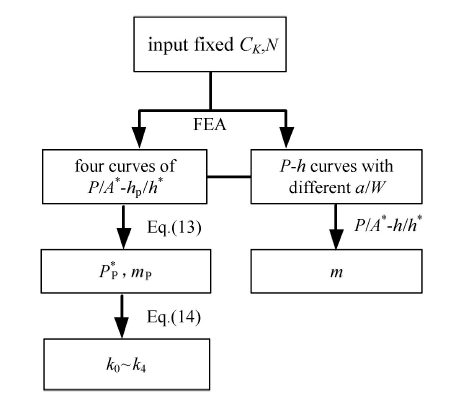Fig. 2   Flow chart of parameters acquisition

Table 1   The size of various cracked components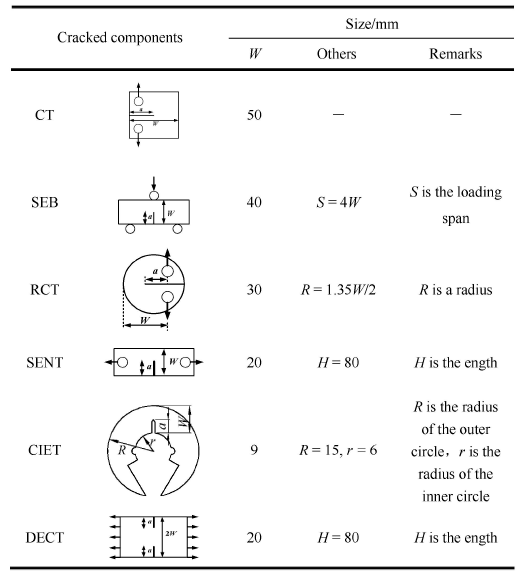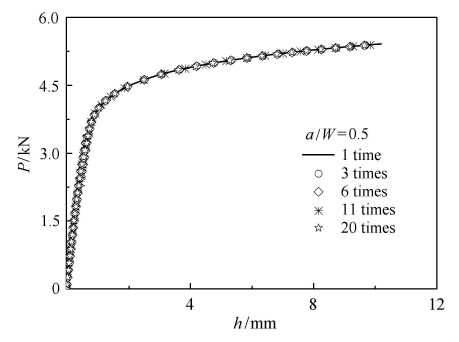Fig. 3   Effect of crack tip grid on load-displacement curves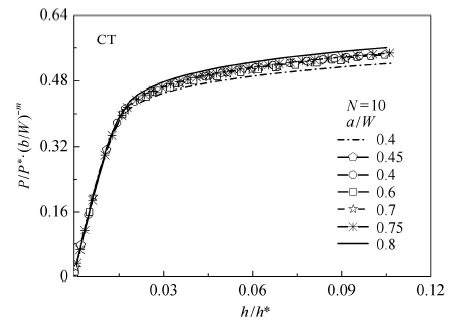Fig. 4   Geometry-independent load-displacement curves of CT specimen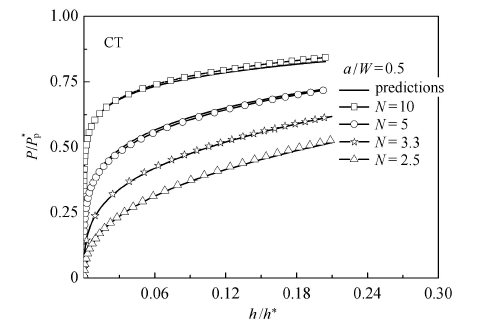Fig. 5   Comparison of fully plastic load-displacement curves predicted by formula and those from FEA for CT specimen

Table 2   Unified formula parameters of J-integral for various cracked components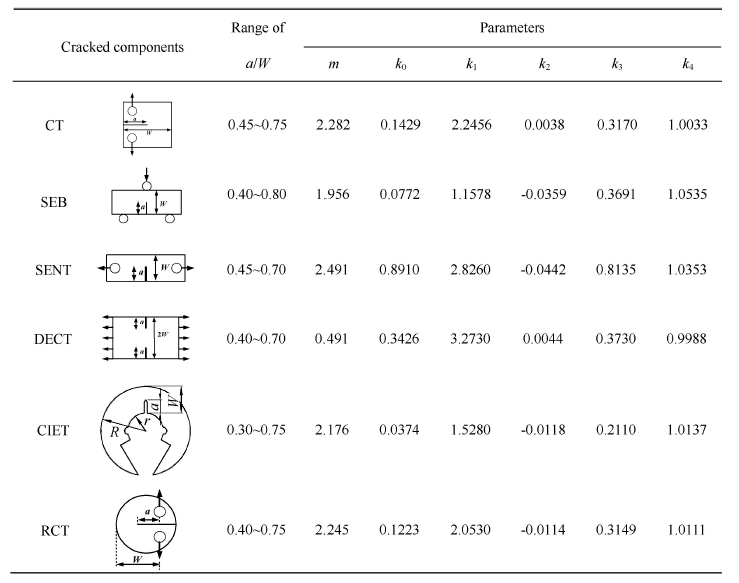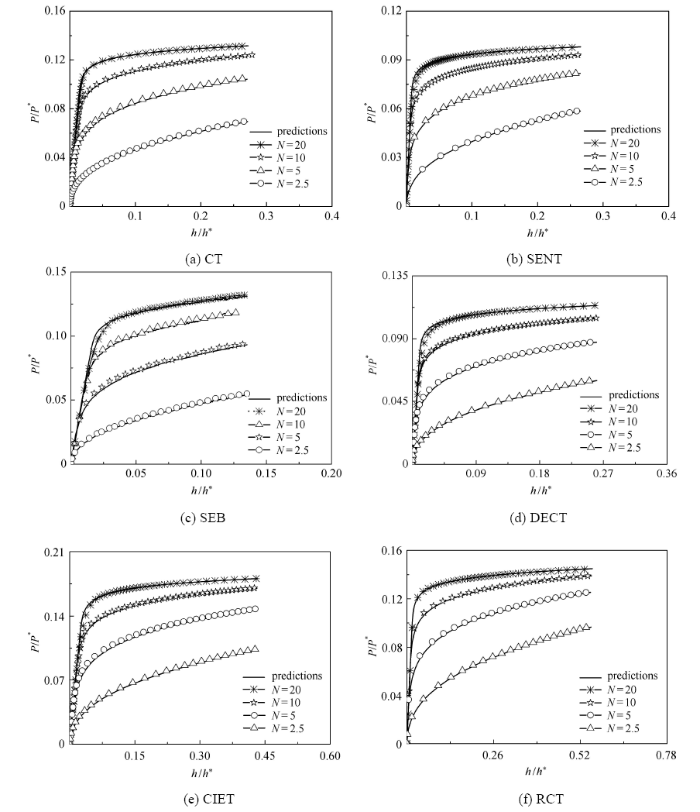Fig.6   Comparison of elastoplastic load-displacement curves predicted by Eq.(19) with those from FEA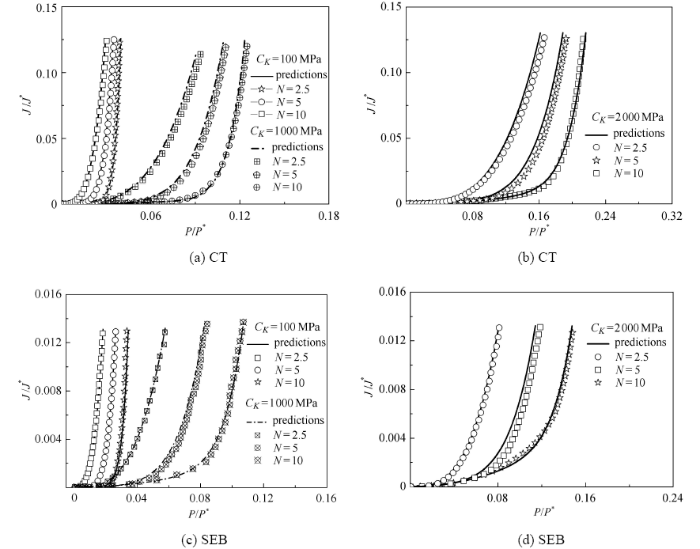Fig. 7   Comparison of J-integral predicted by Eq.(23) with those from FEA

## 3 结 论

(1) 基于Chen-Cai能量等效假设,结合 $J$积分能量定义式,提出了一种解析求解I型裂纹构元 $J$积分的方法, 这种新方法 旨在针对一系列I型裂纹标准试样和非标准试样可以较易实现获得 $J$积分半解析的表达式,以便用于测试和断裂问题的理性分析.

(2)对于6种I型裂纹构元,通过有限元的计算给出了 $J$积分-载荷和载荷-位移统一公式的参数;依靠 $J$积分统一公式预测的 $J$积分结果相较有限元结果都吻合较好.

The authors have declared that no competing interests exist.

## 参考文献 原文顺序 文献年度倒序 文中引用次数倒序 被引期刊影响因子

  胡海岩, 赵永辉, 黄锐. 飞机结构气动弹性分析与控制研究. 力学学报, 2016, 48(1): 1-27 (Hu Haiyan, Zhao Yonghui, Huang Rui.Studies on aeroelastic analysis and control of aircraft structures.Chinese Journal of Theoretical and Applied Mechanics, 2016, 48(1): 1-27 (in Chinese))  柳占立, 庄茁, 孟庆国等. 页岩气高效开采的力学问题与挑战. 力学学报, 2017, 49(3): 507-516 (Liu Zhanli, Zhuang Zhuo, Meng Qingguo, et al.Problems and challenges of mechanics in shale gas efficient exploitation.Chinese Journal of Theoretical and Applied Mechanics, 2017, 49(3): 507-516 (in Chinese))  Kumar V, German MD, Shih CF.Engineering Approach for Elastic-plastic Fracture Analysis. General Electric Co., Schenectady, New York: Corporate Research and Development Dept., 1981  Kumar V, German MDWWW.Development on Elastic-plastic Fracture Analysis (EPRI NP-3607). Palo Alto, California: Research & Development Center of EPRI, 1984  Zahoor A.Ductile Fracture Handbook. vols 1-3, EPRI NP-6301-D/N14- 1, Novetech Corp. and Electric Power Research Institute (Palo Alto, CA), 1989, 1990, 1991  Rice JR.A path independent integral and the approximate analysis of strain concentration by notches and cracks.Journal of Applied Mechanics, 1968, 35(2): 379-386  Zhu XK, Joyce JA.Review of fracture toughness (G, K, J, CTOD, CTOA) testing and standardization.Engineering Fracture Mechanics, 2012, 85: 1-46  嵇醒. 断裂力学判据的评述. 力学学报, 2016, 48(4): 741-753 (Ji Xing.A critical review on criteria of fracture mechanics.Chinese Journal of Theoretical and Applied Mechanics, 2016, 48(4): 741-753 (in Chinese))  GB/T 21143-2014. 金属材料准静态断裂韧度的统一试验方法. 北京:中国标准出版社,2015 (GB/T 21143-2014. Metallic materials-unified method of test for determination of quasistatic fracture toughness. Beijing:Standards Press of China, 2015 (in Chinese))  ASTM E399-09. Standard test methods for linear-elastic plane-strain fracture toughness Klc of metallic materials. Annual Book of ASTM Standards: Vol.3.01. PhiladelPhia, PA: Ameriean Society for Testing and Materials, 2009  ASTM E1820-09. Standard test methods for measurement of fracture toughness// Annual Book of ASTM Standards: Vol.3.01. PhiladelPhia, PA: Ameriean Society for Testing and Materials, 2009  Sun PJ, Wang GZ, Xuan FZ, et al.Three-dimensional numerical analysis of out-of-plane creep crack-tip constraint in compact tension specimens.International Journal of Pressure Vessels and Piping, 2012, 96: 78-89  Traore Y, Paddea S, Bouchard PJ, et al.Measurement of the residual stress tensor in a compact tension weld specimen.Experimental Mechanics, 2013, 53(4): 605-618  Quino G, El Yagoubi J, Lubineau G.Characterizing the toughness of an epoxy resin after wet aging using compact tension specimens with non-uniform moisture content.Polymer Degradation and Stability, 2014, 109: 319-326  Feddern G, Macherauch E.New specimen geometry for KIC measurements. Z.Metallkunde, 1973, 64(12): 882-884  Szkodo M, Bień A. Influence of laser processing of the low alloy medium carbon structural steel on the development of the fatigue crack.Surface and Coatings Technology, 2016, 296: 117-123  Klysz S, Lisiecki J, Leski A, et al.Least squares method modification applied to the NASGRO equation.Journal of Theoretical and Applied Mechanics, 2013, 51(1): 3-13  Chiesa M, Nyhus B, Skallerud B, et al.Efficient fracture assessment of pipelines. A constraint-corrected SENT specimen approach.Engineering Fracture Mechanics, 2001, 68(5): 527-547  Kingklang S, Uthaisangsuk V.Plastic deformation and fracture behavior of X65 pipeline steel: Experiments and modeling.Engineering Fracture Mechanics, 2018, 191: 82-101  Hertelé S, De Waele W, Verstraete M, et al.J-integral analysis of heterogeneous mismatched girth welds in clamped single-edge notched tension specimens.International Journal of Pressure Vessels and Piping, 2014, 119: 95-107  Bao C, Cai LX, Dan C.Estimation of fatigue crack growth behavior for small-sized C-shaped inside edge-notched tension (CIET) specimen using compliance technique.International Journal of Fatigue, 2015, 81: 202-212  但晨, 蔡力勋, 包陈等. 用于断裂韧度测试的C形环小试样的规则化方法与应用. 机械工程学报, 2015, 51(14): 54-65 (Dan Chen, Cai Lixun, Bao Chen, et al.Normalization method used to determine fracture toughness with C-ring small sized specimen and its application.Journal of Mechanical Engineering, 2015,51(14):54-65 (in Chinese))  Treifi M, Oyadiji SO, Tsang DKL.Computations of the stress intensity factors of double-edge and centre V-notched plates under tension and anti-plane shear by the fractal-like finite element method.Engineering Fracture Mechanics, 2009, 76(13): 2091-2108  Bucci RJ, Paris PC, Landes JD, et al.J integral estimation procedures//Fracture Toughness: Part II. ASTM International, 1972  Ernst HA, Paris PC, Landes JD.Estimations on J-integral and tearing modulus T from a single specimen test record//Fracture Mechanics. ASTM International, 1981  Zahoor A.Fracture of circumferentially cracked pipes.Journal of Pressure Vessel Technology, 1986, 108(4): 529-531  Zahoor A.Evaluation of J-integral estimation scheme for flawed throughwall pipes.Nuclear Engineering and Design, 1987, 100(1): 1-9  Goldman NL, Hutchinson JW.Fully plastic crack problems: the center-cracked strip under plane strain.International Journal of Solids and Structures, 1975, 11(5): 575-591  ASTM E1820-15: International. Standard test method for measurement of fracture toughness. ASTM International, 2015  Merkle JG, Corten HT.A J-integral analysis for the compact specimen, considering axial force as well as bending effects. Paper no. 74, PVP 33. American Society for Testing and Materials, 1974  包陈, 蔡力勋, 但晨等.考虑裂尖约束效应的小尺寸 CIET试样延性断裂行为. 机械工程学报, 2017, 53(2): 34-44 (Bao Chen, Cai Lixun, Dan Chen, et al.Ductile fracture behavior of small-sized CIET specimen considering crack front constraint effect.Journal of Mechanical Engineering, 2017, 53(2): 34-44 (in Chinese))  Rice JR.A path independent integral and the approximate analysis of strain concentration by notches and cracks.Journal of Applied Mechanics, 1968, 35(2): 379-386  Chen H, Cai LX.Theoretical model for predicting uniaxial stress-strain relation by dual conical indentation based on equivalent energy principle.Acta Materialia, 2016, 121: 181-189  Chen H, Cai LX.Unified elastoplastic model based on strain energy equivalence principle.Applied Mathematical Modelling, 2017, 52: 664-671  Chen H, Cai LX.Unified ring-compression model for determining tensile properties of tubular materials.Materials Today Communications, 2017, 13: 210-220  Chen H, Cai LX.Theoretical conversions of different hardness and tensile strength for ductile materials based on stress-strain curves.Metallurgical and Materials Transactions A, 2018, 49(4): 1090-1101/

 〈〉